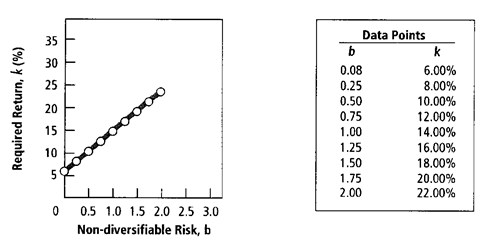.00

# Keywords

Summer 2012/13
ASSIGNMENT

This assignment is to be completed in groups of three and carries 30 per-cent of the marks in this unit.

Part A (15 marks)
Consider the following information
 All Ords Month Index Value Stock A Return Stock B Return D 240.00 J 274.08 0.09570 0.07290 F 284.20 -0.00410 0.08350 M 291.70 0.05420 0.08410 A 288.36 -0.00400 0.06660 M 290.10 -0.03630 -0.00080 J 304.00 0.14640 0.02250 J 318.66 0.09630 -0.00920 A 329.80 0.02030 0.04580 S 318.56 -0.05630 -0.09810 O 320.00 0.03132 0.12740 N 334.16 -0.07770 0.09590 D 380.34 0.09800 0.05280

The risk free rate is 0.02 and you are considering investing 60?f your funds in Stock A and 40?n Stock B.

Calculate the following.

a) Expected Return of Stock A (.5 mark)
b) Expected Return of Stock B (.5 mark)
c) Standard Deviation of Stock A (.5 mark)
d) Standard Deviation of Stock B (.5 mark)
e) Coefficient of Variation of Stock A (.25 mark)
f) Coefficient of Variation of Stock B (.25 mark)
g) Covariance of Stocks A and B (.5 mark)
h) Correlation Coefficient of Stocks A and B (.5 mark)
i) Portfolio Return (.25 mark)
j) Portfolio Standard Deviation and Variance (1.25 mark)
k) Weights of the Minimum Variance Portfolio (1.25 marks)
l) Proof that these weights lead to the Minimum Variance Portfolio (1 mark)
m) Weights of the Optimal Risky Portfolio with a risk-free asset (1 mark)
n) Proof that these weights lead to the Optimal Risky Portfolio (1.25 marks)
o) Discussion on what you would do with this portfolio (1 mark)
p) Calculate the beta for Stock A (1 mark)
q) Using the Excel regression function regress Stock A returns against the market (.5 mark)
r) Calculate the beta for stock B (1 mark)
s) Using the Excel regression function regress Stock B returns against the market (.5 mark)
t)
 Stock A Stock B Ending Stock Price \$ 2.30 Ending Stock Price \$ 4.70 Beginning Stock Price \$ 2.20 Beginning Stock Price \$ 4.45 Anticipated Dividend \$ 0.05 Anticipated Dividend \$ 0.09
Using the estimated returns above determine if either Stock A or Stock B is under/over-priced according to the CAPM (1.5 marks)

Part B (7.5 marks)
Oneway Ltd had outperformed its competition for the past decade however analysts now consider that the company may experience little or no growth in the future. Not perturbed by this the company believes it could maintain a constant annual growth rate in dividends per share of 6 per cent in the future, or possibly 8 per cent for the next two years and 6 per cent thereafter based on estimates of expansion into Asian/pacific markets. By venturing into these markets, the risk of the firm as measured by beta was expected to immediately increase from 1.10 to 1.25.

The chief financial has compiled the following financial data.

 Data item Earnings per share \$ 6.25 Price per share \$ 40.00 Book value of shareholders equity \$ 60 000 000 Total shared issued \$ 2 500 000 Dividend per share \$ 4.00

Figure 1: Security market line for Oncore InternationalRequired

1. Ã¢â‚¬ËœWhat is the firmÃ¢â‚¬â„¢s current price/earnings ratio?
2. Ã¢â‚¬ËœWhat is the firmÃ¢â‚¬â„¢s current book value per share?
3a. What are the required return and risk premium for Oneway LtdÃ¢â‚¬â„¢s shares using the capital asset pricing model (CAPM), assuming the beta of 1.10?
(Hint: Use the security market line - with data points noted - given in Figure 1 to find the market return.)
3b. What are the required return and risk premium for Oneway LtdÃ¢â‚¬â„¢s shares using the (CAPM), assuming the beta of 1.25?
3c. What is the effect on the required return if the beta rises as expected?
4. If the securities analysts are correct and there is no growth in future dividends, what is the value per share of Oneway Ltd? (Note: Beta = 1.25.)
5a. If predictions are correct, what is the value per share of Oneway if the firm maintains a constant annual 6 per cent growth rate in future dividends? (Note: Beta = 1.25.)
5b. If predictions are correct, what is the value per share of Oneway if the firm maintains a constant annual 8 per cent growth rate in dividends per share over the next two years and 6 per cent thereafter? (Note: Beta = 1.25.)
6. Compare the current price of the shares and the share values found in questions 2, 4 and 5. Discuss why these values may differ. Which valuation method do you believe most clearly represents the true value of the Oneway shares?

Part C (7.5 marks)
a) How is a companyÃ¢â‚¬â„¢s cost of capital affected by its tax rate?
b) Explain the common sources of risk affecting financial managers and shareholders
• Risk-Indifferent
• Risk Averse
• Risk Seeking

# Question Set #16

Views: 1456
Word Count: 2083 words including References
File Format: Microsoft Word Document
Editable: Yes

Worksheets: 2 worksheet
File Format: Microsoft Excel Workbook
Editable: Yes